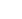# In a study to determine the relationship between two variables, a coefficient of correlation of -0.90 was obtained

In a study to determine the relationship between two variables, a coefficient of correlation of -0.90 was obtained. This indicates: Select one: a. The computations are wrong since coefficient of correlation cannot be negative.b. There is a fairly low relationship between the two variables.c. The coefficient of determination is the square root of 0.90.d. Variable Y tends to decrease as variable X increases.e. The slope in corresponding simple linear regression model must be positive.

Automated page speed optimizations for fast site performance